CalGraph Bob's Calculus## Separating Objects in Space

INTRODUCTION

A recurring problem in computer graphics and robot motion planning is to determine if objects can be separated without colliding. In a video game, one needs to move representations of people and machines without their images overlapping. An explosion can be depicted on screen as a simultaneous separation of particles. One may wish to send a robot through a field of obstacles.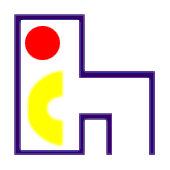There are many variations within this class of problems. Aside from rare examples such as the sofa problem in which one attempts to specify the shape of an object which must follow a specified trajectory, most published problems pertain to moving objects which are restricted to, for example, spheres, isothetic rectangles, smooth convex bodies, convex polygons or star-shaped polygons. The motion is often limited to translations and sometimes only to translations parallel to a given line or set of axes. One might inquire whether a single object can be perturbed without disturbing the others or investigate if an object can be moved as far apart from the others as desired. Some collections of objects can only be separated sequentially, others only if subgroups are first separated and still others only if all are set in motion simultaneously. The answers can vary with the dimension of the space in which the objects reside. By a theorem of Dawson, any collection of more than two smooth convex objects in R2 can be separated, but counter-examples exist to the analogous theorem for R3.

The solutions of the above problems engender many more problems. Having determined theoretically that any collection of a particular class of objects possesses a particular type of separability, one might still need to develop an efficient algorithm to actually perform the motion. For example, after determining that a sphere can be distanced along a line from a set of other spheres if that line does not intersect the contour of the object formed by unifying all the other spheres, it is a not obvious how to mathematically represent that contour. One may wish to determine the shortest path (without collisions) relative to distance or time in directing a robot through a set of obstacles.

DEFINITIONS

Two objects collide if their interiors intersect.

N.B. In all the definitions and theorems below, it will be assumed that, initially the interiors of the objects of any collection are pairwise disjoint. (i.e. Their boundaries may intersect.)

A body B is said to be moveable relative to a collection of bodies {Oa} if it is possible to effect a continuous sequence of rigid motions {f(t): 0<=t<=1} with f(0)=B, f(1) is not= B and for all a and for all t, the interiors f(t) and Oa do not intersect. If, in addition, for all d>0, there exists a continuous family of rigid motions {f(t):0 <= t <= 1} with f(0) = B and distance {f(1), B}>= d, B is said to be moveable to infinity.Moveable objects not moveable to infinity Moveable objects moveable to infinity

A collection of bodies {Bi}1 <= i <= n is movably separable if there exists a sequence of rigid motions such that for each pair of distinct k and l: 1<=k,l<=n, for each d>0 eventually distance {Bk, Bl}>d.

A collection of bodies {Bi}1 <= i <= n is interlocked if it is not movably separable .

A collection of bodies {Bi}1 <= i <= n is sequentially separable if for all k: 1<=k<=n, Bk is moveable to infinity relative to {Bi}-Bk. As the diagram below illustrates, a collection of bodies can be movably separable without being sequentially separable.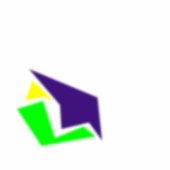Objects sequentially separable Objects moveably separable but not sequentially separable

A collection of bodies {Bi}1 <= i <= n is simultaneously movably separable if it is interlocked but the bodies can still be separated by moving subsets of {Bi}1 <= i <= n simultaneously. (Each such subset includes at least two bodies) The fact that such collections of objects exist is illustrated by the diagram below and our applet.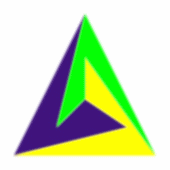Objects simultaneously moveable separable

A collection of rectangles is isothetic if each of their sides is parallel to a coordinate axis.A collection of isothetic rectangles

A polygon P is described as simple if the only points of the plane that belong to exactly two edges are the vertices of P and no points of the plane belong to more than two edges.

A simple polygon is monotone with respect to a line L if for every line m perpendicular to L the intersection of the polygon with m is connected, i.e. the intersection must be a line segment, a point, or empty.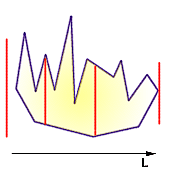Monotone polygon

If we can find a point x0 in a solid body B such that for all x in B, the line joining x0 and x is wholly in B, B is star-shaped about x0. The set of such x0 is called the kernel of B.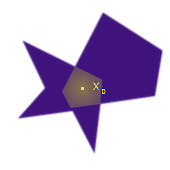Star shaped polygon x0 is one of the points of the kernel. It can see any other point inside of the polygon.

If for all points x and y in a solid body B, the line joining x and y is wholly in B, B is convex. Note that convex bodies are star-shaped about all their points and monotone in every direction.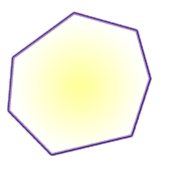Convex polygon

An n-sided simple polygon is star-shaped if n <= 5 and convex if it is a triangle. (The first observation was employed in the design of our applet to ensure that the objects the user creates are star-shaped. Neither assertion admits a converse.)

The convex hull of a set of points is the intersection of all possible convex objects which contain them.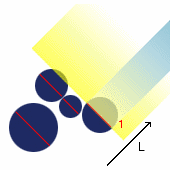Consider a problem of separating circles in sequence using translations along a certain direction L. One of the approaches is to sweep a line perpendicular to L from infinity. The first circle whose center is completely crossed by the sweeping line can be moved to infinity (circle 1 in the picture). The algorithm is then applied to the remaining circles. The fact that the sweeping line crosses the center of a circle guarantees an empty corridor whose width is equal to the circle's diameter.

Next, consider the problem of separating isothetic rectangles along a specified line L. (L need not be parallel to a coordinate axis) A somewhat different approach may be suggested. Construct a line of support (see the diagram below) for each rectangle perpendicular to L. This line of support intersects rectangles A,B,C, and D at the support vertices a,b,c, and d, respectively. One might suggest that an ordered sets of translations can be obtained by sorting the projections of the support vertices on L. It works fine for rectangle B . However, it fails to translate rectangle D, since the latter is blocked by rectangles A and C although the projection of its support vertex comes second in the list. Hence this method does not work.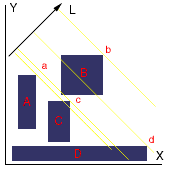Lines of support a,b,c, and d for isothetic rectangles A,B,C, and D respectively

The following method of finding the first rectangle that can be moved in the direction given can also be applied to translating any set of monotonic polygons in a specified direction. Given a set of rectangles and a direction in which they are to be translated consider two sides of each rectangle which intersect at the support vertex. The two line chain formed by these sides consists of 3 vertices. Rotate the axes such that the X-axis is parallel to the direction of translation . The top vertex is the one that has the largest Y-coordinate relative to the direction of translation . Next imagine that the set of chains is illuminated by a light bulb positioned at infinity. We assert that the chain with lowest illuminated top vertex can be moved first in a specified direction. Indeed, were this not true, (i.e. there is another 2-side chain obscuring the selected chain), then that chain would had the lowest illuminated top vertex.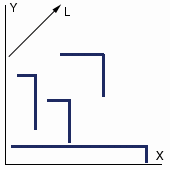To determine the translation ordering of a set of any monotonic polygons, start by isolating a chain of each polygon bounded by the top and bottom vertex (relative to the direction of translation). This can be done by bringing parallel lines of support from plus infinity and minus infinty in the direction perpendicular to L until each line ecounters the first vertex of the polygon on either side. The line sweeping from the top encounter the top vertex, and the one sweeping from the bottom encounters the bottom vertex. These two vertices divide the polygonal chain into two subchains. Consider the subchain whose vertices have a higher X-coordinate relative to L. Illuminate the obtained subchains with a light source coming from infinity parallel to L. The subchain with the lowest illuminated top vertex can be translated in direction L. Proceed in the same manner for the remaining subchains.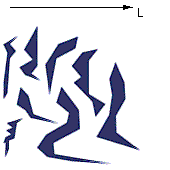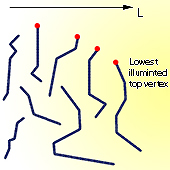A set of polygons to be translated in direction L Illuminated right polygonal chains. The polygon with the lowest illuminated top vertex can be moved in the direction L

Theorem 1 (Robert Dawson 1984)

In any collection of m balls in Rn, there exist at least min{m,n+1} balls which are movable to infinity .The above result was strengthened by Professor Toussaint.

Theorem 1 A (Godfried Toussaint 1984)

Consider a collection of balls {Bi} in Rn. Let h (h <= r) be the number of centres of those balls which lie on either the vertices, edges or faces of the convex hull of {Bi}. Then at least h of the balls are moveable to infinity.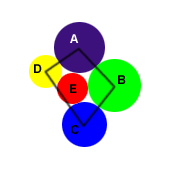Balls A, B, C, and D are moveable to infinity

Theorem 2 (Robert Dawson 1984)

In any collection of at least three smooth convex bodies in R2, at least three elements are movable.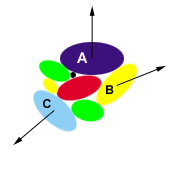Objects A, B, and C are moveable to infinity

This theorem, however, is not necessarily true for R3 . Below is a picture of a convex tile (undergoing rotations around the vertical axis clockwise) which if connected with similar tiles cannot be pushed out or pushed in. There exists a configuration of 12 such tiles, such that there is not a single tile which may be moved without disturbing the others.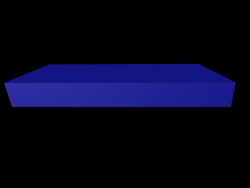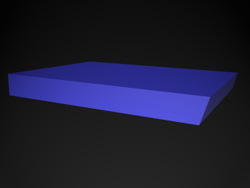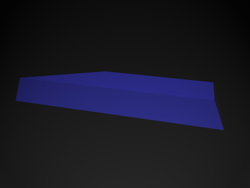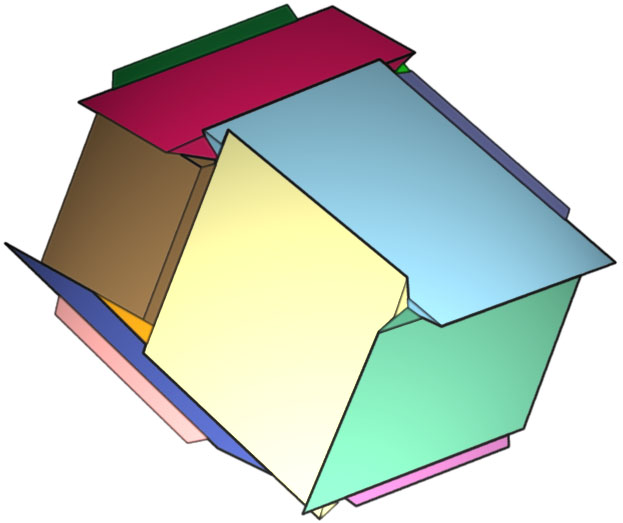Twelve convex tiles, such that there is not one which may be moved without disturbing the others

Theorem 3 (Robert Dawson 1984)

Any collection {Bi}1 <= i <= 1 of star-shaped objects in Rn is simultaneously movably separable.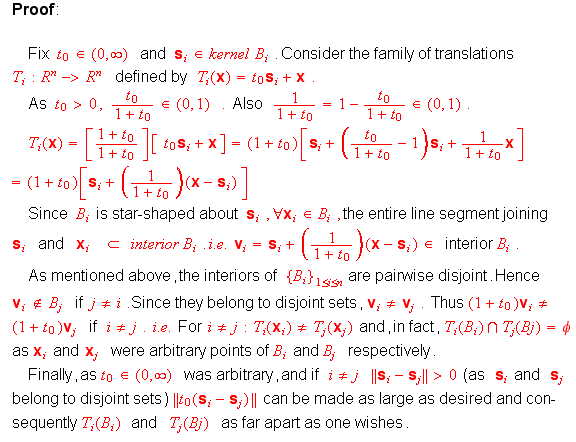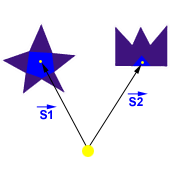EXAMPLES OF PUZZLES (Prof. Toussaint separates objects using a sequence of rotations and translations)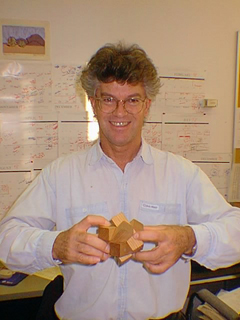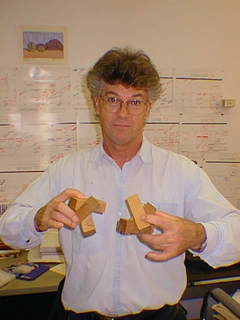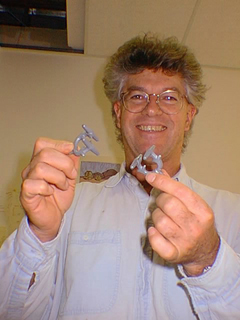REFERENCES

(1) R.J. Dawson, ''On removing a ball without disturbing the others ,'' Mathematics Magazine, vol57, no1, January 1984, pp27-30.

(2) G.T. Toussaint, ''On translating a set of spheres'', Technical Report SOCS-84.4, School of Computer Science, Mcgill University, March 1984.

(3) G.T. Toussaint, ''Moveable Separability of Sets'', Technical Report No. SOCS-84.17, School of Computer Science, Mcgill University, November 1984.